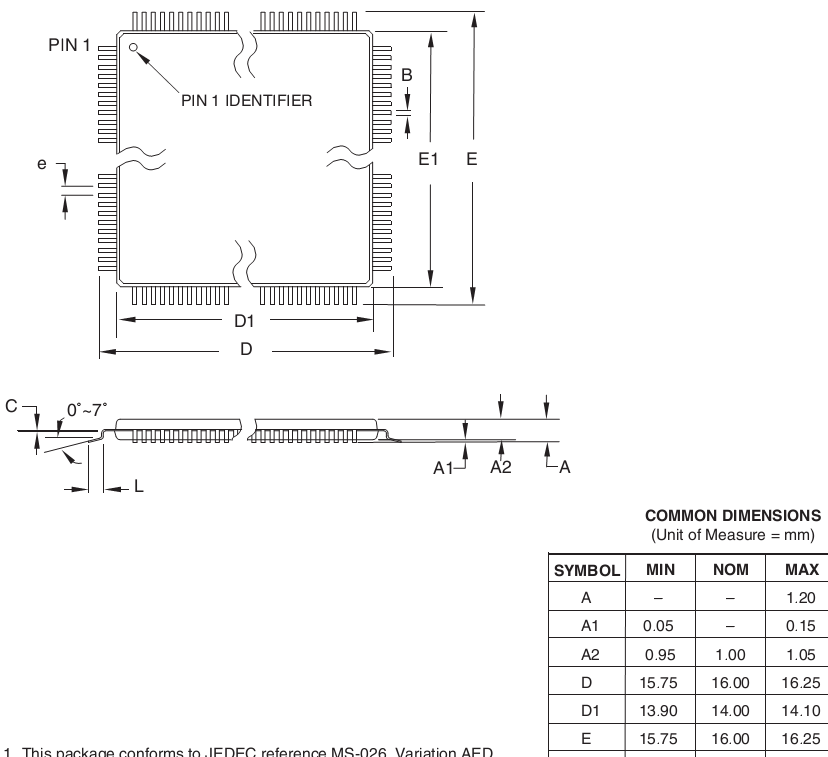## How to calculate distance travelled by Robot in avr

6 posts / 0 new
Author
Message

Here, I want to calculate distance travelled by robot in atmega 2560? I know the formula distance = Wheel's circumference * motor's rotation in rpm. But I am not getting how to apply this formula for the following problem.

```What is the approximate distance covered by the robot in 2 seconds, if OCR5AL=OCR5BL=0xB2. Given that, maximum speed at which the motors rotate is 300 rpm and wheels have a radius of 2.8cm.
Where
OCR5AL is Output compare register low value for Left Motor
OCR5BL is Output compare register low value for Right Motor

Maximum speed is attained, when OCR5AL =OCR5BL =0xFF.```
Last Edited: Sun. Mar 4, 2018 - 01:53 PM

Homework/Classwork question?

#1 This forum helps those that help themselves

#2 All grounds are not created equal

#3 How have you proved that your chip is running at xxMHz?

#4 "If you think you need floating point to solve the problem then you don't understand the problem. If you really do need floating point then you have a problem you do not understand." - Heater's ex-boss

Circumference is 2 * PI * radius

(And if that is still not obvious then slot radius into the above, work out how many revolutions in 2 seconds if 300 revolutions per minute and multiply by that)

Then multiply by ratio of 0xB2 to max OCR

This is the kind of mathematics taught to 10 year olds.

Last Edited: Sun. Mar 4, 2018 - 11:16 AM

clawson wrote:

That said, IME that isn't the way it is done to calibrate bicycles or farm equipment or similar.  At least not the whole answer.  There is the issue of compression of pneumatic tires, as well as slippage.  Generally, one lays out a track and moves the unit for n revolutions under simulated real-world conditions, and then does the calculation.

Partial revolutions are left as an exercise for the student.

Luckily, OP's challenge question gave the assumption of straight-line operation.  (Does the robot have any of the compression and slippage factors?)

sanvipatel wrote:
I want to calculate distance travelled by robot in atmega 2560?
sanvipatel wrote:
distance covered by the robot in 2 seconds

1)  Mark a starting line.

2)  Run robot for two seconds; mark ending point.

3)  Measure the length of ATmega2560.  Or, for "close enough", use datasheet figure.  I'd use 16mm; see below.

4)  Beginning at starting line, repeatedly lay the AVR out in a line to the ending point.  Count how many lengths.  When the ending point is reached multiply the number of AVR lengths by the length of one AVR to get the distance.

4a)  In case 4) above is not understood, obtain a large supply of ATmega2560 and lay then end-to-end from starting line to ending point.  Count the number of AVRs used and multiply by 16mm.https://www.avrfreaks.net/comment...

"how to measure the height of a building with a barometer" joke - you can lower the barometer from the top of the building using rope, then measure the rope; you can give the janitor the barometer if he tells you the height of the building; you can drop the barometer from the top and time how long it takes to hit the bottom; ...

You can put lipstick on a pig, but it is still a pig.

I've never met a pig I didn't like, as long as you have some salt and pepper.

Last Edited: Sun. Mar 4, 2018 - 03:29 PM

Count the number of AVRs used and multiply by 16mm.

Har ..har..har..good one.

Next, you'll probably be cooking a turkey dinner.

When in the dark remember-the future looks brighter than ever.   I look forward to being able to predict the future!